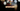# Leetcode | Solution of Reverse String in JavaScript

April 1st, 2020
|

In this post, we will solve reverse string from leetcode and compute it's time and space complexities. Let's begin.

# Problem Statement

The question can be found at leetcode reverse string problem.

The problem states that we need to reverse a string which is in a format of a character array

# Constraints and challenges

The following constraints are given in the question

• We have to do it in O(n) memory

# Solution

We will discuss a solution using two pointer method

We'll take the following approach

• We'll start with two pointers i.e. `left` and `right` which will point to start and end of the array respectively
• We will loop over array till left pointer is less than right pointer and swap the value at both the pointers iteratively

We have discussed the approach, I urge you to go ahead on leetcode and give it another try.If you are here, it means something went wrong in implementation or you are just too lazy. In any case, let's see a simple implementation of the above logic.
``````var reverseString = function (s) {
let left = 0, right = s.length - 1;

while (left < right) {
const temp = s[left];
s[left] = s[right];
s[right] = temp;

left++;
right--;
}
};``````

The solution is very straight forward. It's just the code counterpart of the approach above.

Here are the stats on submission

``````Status: Accepted
Runtime: 100ms
Memory: 46MB``````

## Time and space complexity

### Time complexity

We are looping over the string array once, so time complexity would be O(n).

### Space complexity

We are not using any extra space. So space complexity is constant, O(1).

# Summary

So, we solved the reverse string problem by using two pointer method and calculated the time and space complexities.

I hope you enjoyed solving this question. This is it for this one, complete source code for this post can be found on my Github Repo. Will see you in the next one.